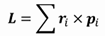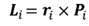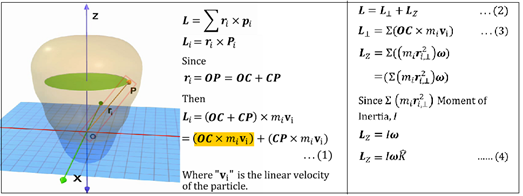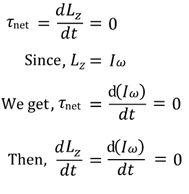Notes On Angular Momentum and Conservation of Angular Momentum - CBSE Class 11 Physics
The angular momentum of a system of particles is denoted byConsider a rigid body rotating about a fixed z-axis. Let the position vector of i-th particle be ri and its linear momentum be pi. Its angular momentum about the origin is denoted byIn general, for a rigid body rotating about a fixed axis, its angular momentum L does not lie along the axis of rotation. It can be shown that for a rigid body that is symmetrical about the axis of rotation, the angular momentum is along its axis of rotation. If the external torque is zero, thenTherefore, we get Lz or Iω as constant. This is the Law of Conservation of Angular Momentum pertaining to rotation about a fixed axis. It states that in the absence of net external torque, the total angular momentum of the system remains conserved.

#### Summary

The angular momentum of a system of particles is denoted byConsider a rigid body rotating about a fixed z-axis. Let the position vector of i-th particle be ri and its linear momentum be pi. Its angular momentum about the origin is denoted byIn general, for a rigid body rotating about a fixed axis, its angular momentum L does not lie along the axis of rotation. It can be shown that for a rigid body that is symmetrical about the axis of rotation, the angular momentum is along its axis of rotation. If the external torque is zero, thenTherefore, we get Lz or Iω as constant. This is the Law of Conservation of Angular Momentum pertaining to rotation about a fixed axis. It states that in the absence of net external torque, the total angular momentum of the system remains conserved.
Previous
Next